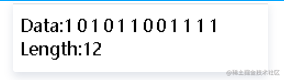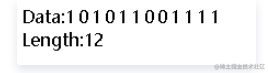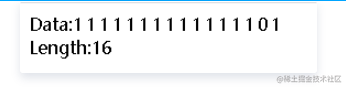# 用c#语言解释二进制源码和补码

## 二进制

#### 无符号数

``````UInt16 decimals = 2767;
string binary = Convert.ToString(decimals,2);
Response.Write("Data:<span>" + binary + "</span><br/>");
Response.Write("Length:"+binary.Length);## 有符号数

`````` Int16 decimals = 2767;
string binary = Convert.ToString(decimals,2);
Response.Write("Data:<span>" + binary + "</span><br/>");
Response.Write("Length:"+binary.Length);## 负数的情况

``````Int16 decimals = -3;
string binary = Convert.ToString(decimals,2);
Response.Write("Data:<span>" + binary + "</span><br/>");
Response.Write("Length:"+binary.Length);